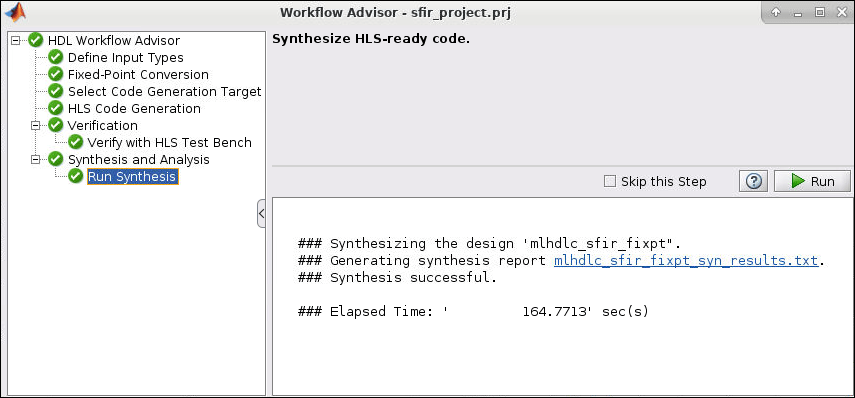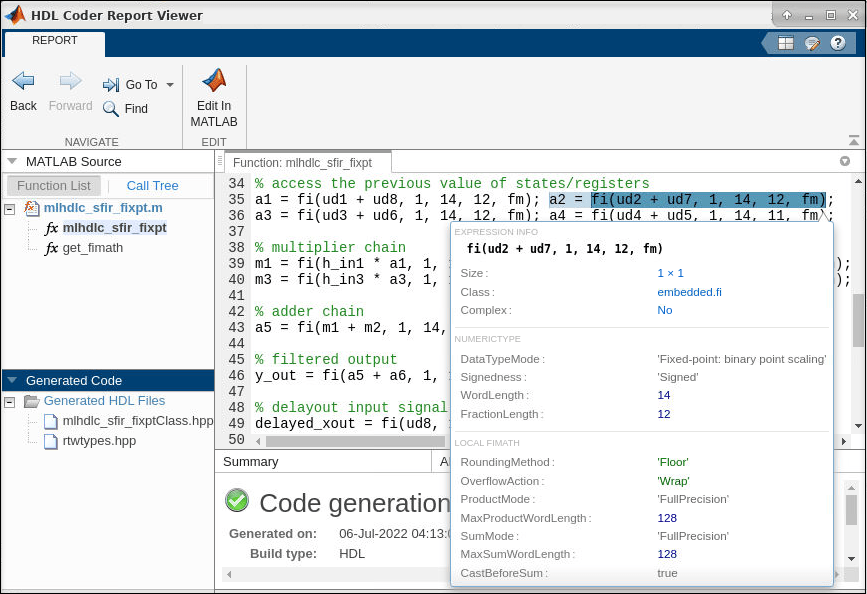# Get Started with MATLAB to SystemC Workflow Using HDL Coder App

This example shows how to create an HDL Coder™ project and generate SystemC code from a MATLAB® design for a symmetric finite impulse response (FIR) filter.

Set up the FIR model and test bench for this example.

```mlhdlc_demo_setup('sfir'); ```

### FIR Filter MATLAB Design

The MATLAB design `mlhdlc_sfir` is a simple symmetric FIR filter.

```design_name = 'mlhdlc_sfir'; testbench_name = 'mlhdlc_sfir_tb'; ```

Review the design.

```open(design_name); ```
```%%%%%%%%%%%%%%%%%%%%%%%%%%%%%%%%%%%%%%%%%%%%%%%%%%%%%%%%%%%%%%%%%%%%%%%%%% % MATLAB design: Symmetric FIR Filter % % Introduction: % % We can reduce the complexity of the FIR filter by leveraging its symmetry. % Symmetry for an n-tap filter implies, coefficient h0 = coefficient hn-1, % coefficient, h1 = coefficient hn-2, etc. In this case, the number of % multipliers can be approximately halved. The key is to add the % two data values that need to be multiplied with the same coefficient % prior to performing the multiplication. % % Key Design pattern covered in this example: % (1) Filter states represented using the persistent variables % (2) Filter coefficients passed in as parameters % Copyright 2011-2019 The MathWorks, Inc. %#codegen function [y_out, delayed_xout] = mlhdlc_sfir(x_in,h_in1,h_in2,h_in3,h_in4) % Symmetric FIR Filter % declare and initialize the delay registers persistent ud1 ud2 ud3 ud4 ud5 ud6 ud7 ud8; if isempty(ud1) ud1 = 0; ud2 = 0; ud3 = 0; ud4 = 0; ud5 = 0; ud6 = 0; ud7 = 0; ud8 = 0; end % access the previous value of states/registers a1 = ud1 + ud8; a2 = ud2 + ud7; a3 = ud3 + ud6; a4 = ud4 + ud5; % multiplier chain m1 = h_in1 * a1; m2 = h_in2 * a2; m3 = h_in3 * a3; m4 = h_in4 * a4; % adder chain a5 = m1 + m2; a6 = m3 + m4; % filtered output y_out = a5 + a6; % delayout input signal delayed_xout = ud8; % update the delay line ud8 = ud7; ud7 = ud6; ud6 = ud5; ud5 = ud4; ud4 = ud3; ud3 = ud2; ud2 = ud1; ud1 = x_in; end ```

### FIR Filter MATLAB Test Bench

The MATLAB test bench `mlhdlc_sfir_tb` tests the filter design. Review this test bench.

```open(testbench_name); ```
```%%%%%%%%%%%%%%%%%%%%%%%%%%%%%%%%%%%%%%%%%%%%%%%%%%%%%%%%%%%%%%%%%%%%%%%%%% % MATLAB test bench for the FIR filter %%%%%%%%%%%%%%%%%%%%%%%%%%%%%%%%%%%%%%%%%%%%%%%%%%%%%%%%%%%%%%%%%%%%%%%%%% % Copyright 2011-2019 The MathWorks, Inc. clear mlhdlc_sfir; T = 2; dt = 0.001; N = T/dt+1; sample_time = 0:dt:T; df = 1/dt; sample_freq = linspace(-1/2,1/2,N).*df; % input signal with noise x_in = cos(2.*pi.*(sample_time).*(1+(sample_time).*75)).'; % filter coefficients h1 = -0.1339; h2 = -0.0838; h3 = 0.2026; h4 = 0.4064; len = length(x_in); y_out = zeros(1,len); x_out = zeros(1,len); for ii=1:len data = x_in(ii); % call to the design 'mlhdlc_sfir' that is targeted for hardware [y_out(ii), x_out(ii)] = mlhdlc_sfir(data, h1, h2, h3, h4); end figure('Name', [mfilename, '_plot']); subplot(3,1,1); plot(1:len,x_in,'-b'); xlabel('Time (ms)') ylabel('Amplitude') title('Input Signal (with noise)') subplot(3,1,2); plot(1:len,y_out,'-b'); xlabel('Time (ms)') ylabel('Amplitude') title('Output Signal (filtered)') freq_fft = @(x) abs(fftshift(fft(x))); subplot(3,1,3); semilogy(sample_freq,freq_fft(x_in),'-b'); hold on semilogy(sample_freq,freq_fft(y_out),'-r') hold off xlabel('Frequency (Hz)') ylabel('Amplitude (dB)') title('Input and Output Signals (Frequency domain)') legend({'FilterIn', 'FilterOut'}, 'Location','South') axis([-500 500 1 100]) ```

### Test MATLAB Algorithm

To avoid run-time errors, simulate the design by using the test bench.

```mlhdlc_sfir_tb ```### Generate SystemC Code by Using HDL Workflow Advisor

SystemC code generation with the HDL Workflow Advisor has the following basic steps:

1. At the MATLAB command line, set up the high-level synthesis (HLS) tool path for SystemC code generation by using the function `hdlsetuphlstoolpath`.

2. Create a MATLAB HDL Coder project.

3. Add the design and test bench files to the project.

4. Start the HDL Workflow Advisor for the MATLAB design.

5. Run fixed-point conversion and SystemC code generation.

### Create HDL Coder Project and Assign Files

To create an HDL Coder project:

1. In the MATLAB Editor, on the Apps tab, select HDL Coder. Enter `sfir_project` as the Name of the project.

To create a project at the MATLAB command prompt, run the command:

```coder -hdlcoder -new sfir_project ```

A `sfir_project.prj` file is created in the current folder.

2. For MATLAB Function, click the Add MATLAB function link and select the FIR filter MATLAB design `mlhdlc_sfir`. Under the MATLAB Test Bench section, click Add files and add the MATLAB test bench `mlhdlc_sfir_tb.m`.

3. Click Autodefine types and use the recommended types for the MATLAB design. The code generator infers the input types from the MATLAB test bench.### Run Fixed-Point Conversion and SystemC Code Generation in HDL Workflow Advisor

1. To start the HDL Workflow Advisor, click the Workflow Advisor button.

2. In HDL Workflow Advisor step, specify Code Generation workflow as MATLAB to SystemC.3. If your design does not use fixed-point types and functions, then translate your floating-point MATLAB design to a fixed-point design. To examine the generated fixed-point code from the floating-point design, click the Fixed-Point Conversion task. The generated fixed-point MATLAB code opens in the MATLAB editor. For details, see Floating-Point to Fixed-Point Conversion.

4. In Select Code Generation Target step, specify Workflow as High Level Synthesis and Synthesis tool as Cadence Stratus.5. Right-click the SystemC Code Generation task and select Run to selected task.

The code generator runs the Workflow Advisor tasks to generate SystemC code for the filter design.

To examine the generated SystemC code, click the hyperlink to `mlhdlc_sfir_fixptClass.hpp` in the SystemC Code Generation log window.6. You can compare the output of the generated SystemC code to that of the MATLAB code in the Verify with HDL Test Bench step. This step also generates SystemC test bench files and creates the Cadence Stratus project.7. Synthesis is the final step in the code generation process. In the Run Synthesis step, the generated SystemC code is synthesized using the HLS tool and Verilog or VHDL code is generated.### SystemC Code Generation Report

At the SystemC Code Generation step, you can check the code generation report by clicking `View Report` in the log window.

The code generation report helps you to:

• Debug code generation issues and verify that your MATLAB code is suitable for code generation.

• View generated SystemC code.

• Access additional reports like a conformance report.

• See how the code generator determines and propagates type information for variables and expressions in your MATLAB code.

To view a MATLAB function in the code pane, click the name of the function in the MATLAB Source pane. In the code pane, when you pause on a variable or expression, a tooltip displays information about its size, type, and complexity.### Limitations

• Only Cadence Stratus is supported as the high level synthesis tool. Cadence Stratus supports only point to point (p2p) communication for SystemC code generation.

• Generating SystemC code that runs on multiple threads is not supported.

• Real data types and Systems objects are not supported for SystemC code generation.

• Structures and enumerations are not supported as inputs and outputs at the top-level DUT ports for SystemC code generation.